Courses

# Test: Mental Ability, Analytical & Logical Reasoning - 7 (2015-2014)

## 20 Questions MCQ Test UPSC Topic Wise Previous Year Questions | Test: Mental Ability, Analytical & Logical Reasoning - 7 (2015-2014)

Description
This mock test of Test: Mental Ability, Analytical & Logical Reasoning - 7 (2015-2014) for UPSC helps you for every UPSC entrance exam. This contains 20 Multiple Choice Questions for UPSC Test: Mental Ability, Analytical & Logical Reasoning - 7 (2015-2014) (mcq) to study with solutions a complete question bank. The solved questions answers in this Test: Mental Ability, Analytical & Logical Reasoning - 7 (2015-2014) quiz give you a good mix of easy questions and tough questions. UPSC students definitely take this Test: Mental Ability, Analytical & Logical Reasoning - 7 (2015-2014) exercise for a better result in the exam. You can find other Test: Mental Ability, Analytical & Logical Reasoning - 7 (2015-2014) extra questions, long questions & short questions for UPSC on EduRev as well by searching above.
QUESTION: 1

### Two men, Anil and David, and two women, Shabnam and Rekha are in a sales group. Only two speak Tamil. The other two speak Marathi. Only one man and one woman can drive a car. Shabnam speaks Marathi. Anil speaks Tamil. Both Rekha and David can drive. Which of the following statements is true? 

Solution: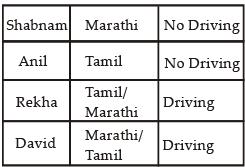From above table, it can be concluded that only of those who can drive car can speaks Tamil.

QUESTION: 2

### A, B, C, D, E and F are cousins. No two cousins are of the same age, but all have birthdays on the same day of the same month. The youngest is 17 years old and the oldest E is 22 years old. F is somewhere between B and D in age. A is older than B. C is older than D. A is one year older than C. What is the number of logically possible orders of all six cousins in terms of increasing age? 

Solution: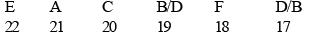Two logically possible order of all six cousins in terms of increasing age.

QUESTION: 3

### A, B, C, D, E and F are cousins. No two cousins are of the same age, but all have birthdays on the same day of the same month. The youngest is 17 years old and the oldest E is 22 years old. F is somewhere between B and D in age. A is older than B. C is older than D. A is one year older than C. Which one of the following is possible? 

Solution:F is 18 years old.

QUESTION: 4

Usha runs faster than Kamala, Priti runs slower than Swati, Swati runs slower than Kamala. Who is the slowest runner?



Solution:

According to question,
Priti < Swati < Kamala < Usha
From the above, it can concluded that priti is the slowest runner.

QUESTION: 5

If ABC × DEED = ABCABC; where A, B, C, D and E are different digits, what are the values of D and E?



Solution:

ABC × DEED = ABCABC
We know that, when we write any Number in form of ABCABC then that number must be divisible by 13 , 11 and 7 or ABCABC  is multiple of 1001
So, 1001 = DEED
1001 = 13 × 11 × 7
ABC × DEED = ABC ABC
⇒ ABC × 1001 = ABC ABC
On comparing
D = 1, E = 0

QUESTION: 6

Consider the following statements:
1. Some claim to have seen UFOs (Unidentified Flying Objects).
2. Life on other heavenly bodies is considered to be a possibility.
3. Voyage to space is now an established fact.
From the above statements, it may be concluded that



Solution:

UFOs may or may not be heavenly bodies. Possibility of life on other heavenly bodies does not prove either that UFOs are heavenly bodies or that they are sent from other heavenly bodies. So, this also cannot be proved that living species in other heavenly bodies are more intelligent than man. Therefore, nothing definite can be said about the UFOs.

QUESTION: 7

Consider the following statements followed by two conclusions: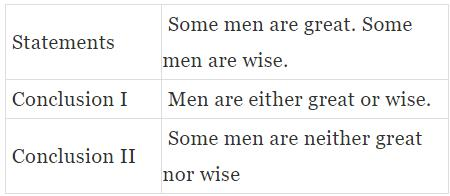Which one of the following is correct?



Solution: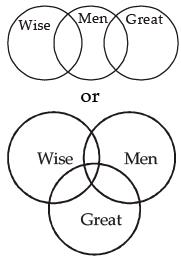Conclusion I : False
Conclusion II : False

QUESTION: 8

Each of the six different faces of a cube has been coated with a different colour i.e., V, I, B, G, Y and O. Following information is given:
1. Colours Y, O and B are on adjacent faces.
2. Colours I, G and Y are on adjacent faces.
3. Colours B, G and Y are on adjacent faces.
4. Colours O, V and B are on adjacent faces.
Which is the colour of the face opposite to the face coloured with O?



Solution:

From 2 and 3, I and B are on opposite faces. from 1 and 4, Y and V are adjacent to O.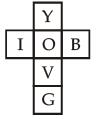Hence, G is the colour of the face opposite to the face coloured with O.

QUESTION: 9

"Liberty, therefore, is never real unless the Government can be called to account when it invades rights."

Which one of the following is the best justification of the above statement?



Solution:

The statement implies that actual liberty is when the ruling government can also be called to account or tried in a court of law when it invades rights.

QUESTION: 10

Price is not the same thing as value. Suppose that on a day the price of everything viz., coal, bread, postage stamps, a day's labour, the rent of houses, etc. were to double. Prices then would certainly rise, but values of all things except one would not."
The writer wants to say that if prices of all things were doubled



Solution:

Due to inflation the value of money only would be halved.

QUESTION: 11

Consider the following figures :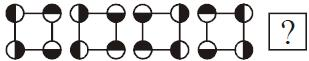Change in positions of beads in the four figures above follows a sequence. Following the same sequence, which of the figures below should appear as the fifth figure above?



Solution:

From 1 to 2 - vertical interchange of half shaded circle.

QUESTION: 12

With reference to the figure given below, the number of different routes from S to T without retracing from U and/or V, is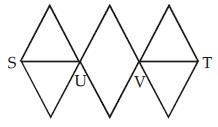Solution: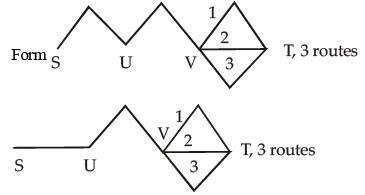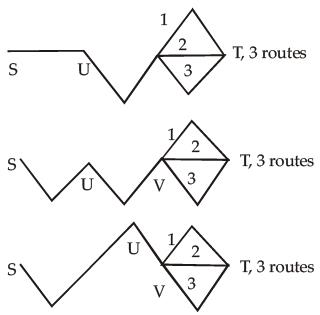Total routes = 3 + 3 + 3 + 3 + 3 + 3 = 18

QUESTION: 13

Consider the following matrix with one empty block in the lower extreme corner :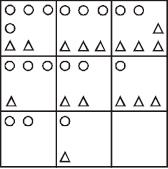Which of the following figures could fit in the empty block and thus complete the matrix?



Solution:

Remove one circle, a triangle is remove,
So,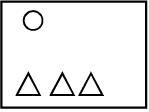after removing circle, triangle is also remove.
So,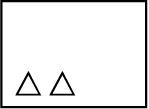A circle and a triangle is reducing by one.

QUESTION: 14

Consider the table given below in which the numbers bear certain relationship among themselves along the rows :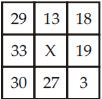Which one of the following numbers is the missing number indicated above by X?



Solution:

Sum of numbers in 1st Row, 29 + 13 + 18 ⇒ 60
Sum of numbers in 3rd Row, 30 + 27 + 3 ⇒ 60
So, 2nd Row, 33 + X + 19 = 60
X + 52 = 60
X = 60 – 52
X = 8

QUESTION: 15

Consider the following matrix :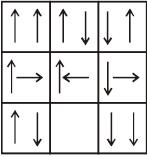Which one of the following figures fits into the blank part of the above matrix?



Solution: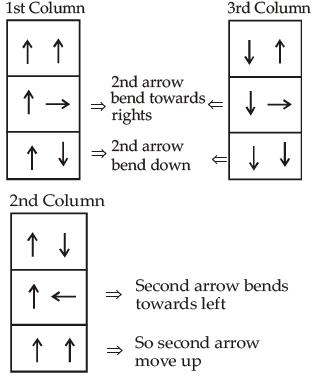QUESTION: 16

Examine the following figure :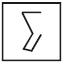Which one of the following figures has the above figure embedded in it?



Solution: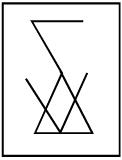QUESTION: 17

Assume that
1. the hour and minute hands of a clock move without jerking.
2. the clock shows a time between 8 o'clock and 9 o'clock.
3. the two hands of the clock are one above the other.
After how many minutes (nearest integer) will the two hands be again lying one above the other?



Solution:

Two hands of clock are on one above between 8 to 9.
12 to 8 ⇒ 40 min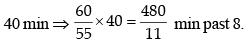two hands of clock are on one above between 9 to 10.
12 to 9 ⇒  45 min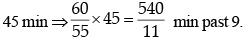So two hands be lying one above the other =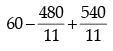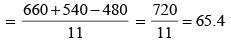QUESTION: 18

Four children are sitting in a row. A is occupying the seat next to B but not next to C. If C is not sitting next to D, who is/are occupying seat/seats adjacent to D?



Solution: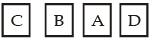Hence, A occupy seats adjacent to D.

QUESTION: 19

Consider the following statements :
There are six villages A, B, C, D, E and F.
F is 1 km to the west of D.
B is 1 km to the east of E.
A is 2 km to the north of E.
C is 1 km to the east of A.
D is 1 km to the south of A.
Which three villages are in a line?



Solution: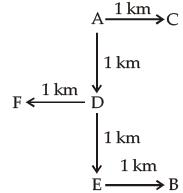Hence, A, D, E in a line.

QUESTION: 20

Consider that :
1. A is taller than B.
2. C is taller than A.
3. D is taller than C.
4. E is the tallest of all.
If they are made to sit in the above order of their height, who will occupy the mid position?



Solution:

Sequence according to height.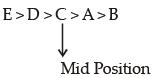Therefore, ‘C’ occupy the mid position.